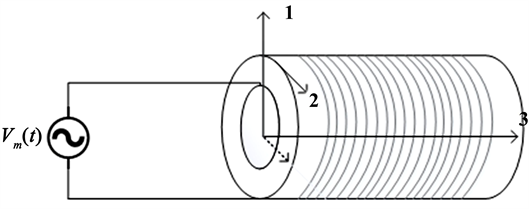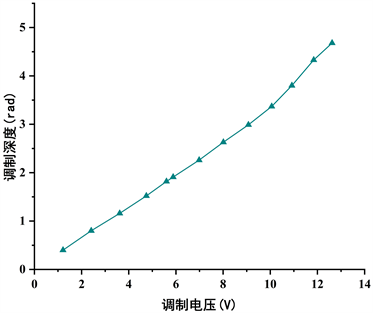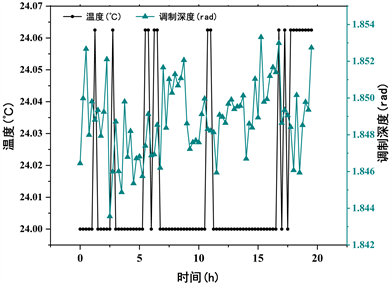# PZT光纤相位调制器调制特性研究Modulation Characteristics of PZT Optical Fiber Phase Modulator

DOI: 10.12677/JSTA.2021.91003, PDF, HTML, XML, 下载: 88  浏览: 1,543  国家科技经费支持

Abstract: PZT (Lead Zirconate Titanate Piezoelectric Ceramics) has good piezoelectric properties after polari-zation. A phase modulator composed of PZT piezoelectric ceramics and optical fibers wound on PZT can achieve phase modulation through the Inverse Piezoelectric Effect. Compared with the com-monly used lithium niobate (LiNbO3) phase modulator, PZT has the advantages of low cost and low access system loss. A theoretical analysis of the modulation principle of the PZT phase modulator is carried out, and the relationship between the PZT drive voltage and the amount of phase change and modulation depth is derived. A test prototype was built based on the open-loop fiber optic cur-rent sensor (FOCS), which verified the excellent modulation performance and temperature stability of the PZT phase modulator and provided theoretical guidance for the sensitivity improvement, op-timization design, and application of the PZT.

1. 引言

PZT在极化后具有良好的压电性能，其压电系数大、居里温度高、各项机电参数受温度影响小、时间稳定性好、易于集成   ，加上本身材质坚硬，不受外界恶劣环境影响等优点  ，常用于光纤相位调制器的制作，以实现信息在光纤中的传输  。

PZT压电陶瓷通过逆压电效应实现相位的调制  。逆压电效应是指在陶瓷管上施加一个与极化方向相同或者相反的电场，会使陶瓷管产生极化方向上的伸缩形变的现象   。开环光纤电流传感器是PZT压电陶瓷作为相位调制器的典型应用。原理图如图1所示：Figure 1. Principle diagram of FOCS

2. PZT相位调制机理

2.1. 加载电压与相位变化

PZT相位调制器通过在PZT上施加调制电压产生逆压电效应，使PZT发生伸缩形变，引起缠绕在PZT管上的光纤长度、纤芯直径和折射率的变化,使在光纤中传输的光波发生相位变化，进而实现相位调制的目的，其示意图如图2所示。Figure 2. PZT phase modulation

$\Delta l\left(t\right)=\frac{{d}_{11}l{V}_{m}\left(t\right)}{{D}_{O}-{D}_{I}}\cdot \frac{{w}_{0}^{2}}{\sqrt{{\left({w}_{0}^{2}-{w}_{m}^{2}\right)}^{2}+{\left(C{w}_{0}{w}_{m}\right)}^{2}}}$ (1)

$\Delta {\phi }_{{n}_{\epsilon z}}=2\pi \left(\Delta n+\Delta {n}_{\epsilon z}\right){\epsilon }_{z}/\lambda$ (2)

$\Delta {n}_{st}=-\frac{CE{\epsilon }_{z}}{1-V}{\int }_{0}^{1}\frac{1}{\pi }{\int }_{0}^{2\pi }\gamma \left(r,\theta \right)\mathrm{cos}2\theta \text{d}\theta \left({r}^{-1}-3{r}^{3}\right)\text{d}r$ (3)

$\Delta {n}_{st}=\frac{2\pi \Delta {n}_{st}l}{\lambda }=\frac{2\pi l{k}_{st}{\epsilon }_{z}}{\lambda }$ (4)

$\Delta \phi =\Delta {\phi }_{{\epsilon }_{Z}}+\Delta {\phi }_{st}$ (5)

$\Delta \phi =\frac{2\pi }{\lambda }\left(\Delta n\cdot \Delta L+L\cdot \Delta {n}_{st}\right)=\frac{2\pi }{\lambda }\left(\Delta n+L\cdot {k}_{st}\right)\Delta L$ (6)

$\Delta \phi =\frac{4N{d}_{11}{\pi }^{2}}{\left({D}_{O}-{D}_{I}\right)\lambda }\left(\Delta n+2\pi {D}_{O}N{k}_{st}\right)\cdot {V}_{m}$ (7)

2.2. 相位变化与调制深度

$\phi \left(t\right)={K}_{m}{V}_{m}\left(t\right)={K}_{m}{A}_{mp}\mathrm{sin}\left({\omega }_{m}t\right)={\phi }_{m0}\mathrm{sin}\left({\omega }_{m}t\right)$ (8)

${P}_{D}=k{P}_{0}\left\{1+\mathrm{cos}\left[{\phi }_{F}\left(t\right)+2{\phi }_{m0}\mathrm{sin}\frac{{\phi }_{m}}{2}\mathrm{cos}{\omega }_{m}\left(t-\frac{\tau }{2}\right)\right]\right\}$ (9)

${\phi }_{md}=2{\phi }_{m0}\mathrm{sin}\frac{{\phi }_{m}}{2}$ (10)

${P}_{D}=k{P}_{0}\left\{1+\mathrm{cos}\left[{\phi }_{F}\left(t\right)+{\phi }_{md}\mathrm{cos}{\omega }_{m}\left(t-\frac{\tau }{2}\right)\right]\right\}$ (11)

3. 试验和分析

3.1. PZT的调制性能试验Figure 3. The relation curve of the voltage applied on the PZT and modulation depth

3.2. PZT调制性能的温度特性试验Figure 4. The modulation characteristics of PZT at a room temperatureFigure 5. The curve of the modulation depth of PZT, when FOCS is in a high-low temperature cycle

4. 结语

NOTES

*通讯作者。

  Panda, P.K., Sahoo, B., Ramakrishna, J., Manoj, B. and Prasada Rao, D.S.D. (2018) Recent Studies on Vibrational En-ergy Harvesting of PZT Materials. Materials Today: Proceedings, 5, 21512-21516. https://doi.org/10.1016/j.matpr.2018.06.562  Wang, R.Z., Tang, E.L., Yang, G.L. and Han, Y.F. (2020) Ex-perimental Research on Dynamic Response of PZT-5H under Impact Load. Ceramics International, 46, 2868-2876. https://doi.org/10.1016/j.ceramint.2019.09.280  程沁蕊. 基于PZT相位调制实时补偿的全光纤傅里叶变换光谱仪建模与设计[D]: [硕士学位论文]. 天津: 天津大学, 2018.  王廷云. 用压电陶瓷实现的光纤相位调制理论与试验分析[J]. 光子学报, 1999(2): 3-5.  Gao, B., Yao, Z.H., Lai, D.Y., et al. (2020) Unexpectedly High Pi-ezoelectric Response in SM-Doped PZT Ceramics beyond the Morphotropic Phase Boundary Region. Journal of Alloys and Compounds, 836, Article ID: 155474. https://doi.org/10.1016/j.jallcom.2020.155474  Liu, C.Y., Xu, P., Zheng, D.Y. and Liu, Q.B. (2020) Study on Microstructure and Properties of a Fe-Based SMA/PZT Composite Coating Produced by Laser Cladding. Journal of Al-loys and Compounds, 831, Article ID: 154813. https://doi.org/10.1016/j.jallcom.2020.154813  任仲豪, 周慎杰. 薄膜压电材料压电系数测试方法研究进展[J]. 传感器与微系统, 2020, 39(10): 1-4.  Kahn, V. (1985) Acoustic and Elastic Properties of PZT Ceramics with Anisotropic Pores. Journal of the American Ceramic Society, 68, 623-628. https://doi.org/10.1111/j.1151-2916.1985.tb16165.x  褚涛, 王安玖, 张元松, 何婧, 王学杰, 张静, 张珍宣, 张田才. 高压电常数PZT-PNN-PSN-PMW压电陶瓷的制备及性能研究[J]. 功能材料与器件学报, 2020, 26(3): 213-218.  杜春雷. 压电陶瓷材料研究现状及其应用[J]. 江西建材, 2020(5): 4+6.  莫国伟. PZT8与PZT4压电陶瓷材料的性能对比[J]. 造纸装备及材料, 2020, 49(3): 59.  金锋, 姬文越. 对光纤PZT相位调制器的研究[J]. 光通信技术, 1989(1): 84-87.  袭超. 光纤干涉式传感系统中PZT调制器的特性研究[D]: [硕士学位论文]. 哈尔滨: 哈尔滨工程大学, 2012.  刘相果, 蹇胜勇, 蒋洪平, 刘光聪. 基于PZT陶瓷谐振技术的光纤相位调制器研究[J]. 压电与声光, 2011, 33(5): 699-701.  杨远洪, 张惟, 叙马静. 开环FOG中PZT调制器的在线研究[J]. 北京航空航天大学学报, 1999(6): 735-738.  杨远洪, 马静, 张惟叙. 光纤陀螺中PZT调制器的建模[J]. 航空精密制造技术, 1996(1): 38-41.  刘志波, 李阳, 苏耘, 刘艳, 谭中伟, 马林, 简水生. 基于压电陶瓷的光相位调制器的调制系数测量及验证[J]. 光学学报, 2013, 33(7): 83-88.  王巍, 杨清生. 全光纤陀螺及其相位调制器设计[J]. 航天控制, 1995(2): 16-21.  胡科可. 光纤干涉信号的数字处理方法的研究[D]: [硕士学位论文]. 杭州: 浙江大学, 2014.  毛献辉. 环形干涉光纤传感器若干关键问题研究[D]: [博士学位论文]. 北京: 清华大学, 2005.  卢成杰. 开环光纤陀螺数字解调关键问题的研究[D]: [硕士学位论文]. 杭州: 浙江大学, 2019.  张敏杰. 开环Sagnac干涉仪的数字信号处理[D]: [硕士学位论文]. 杭州: 浙江大学, 2016.  肖浩, 刘博阳, 湾世伟, 赵玉才. 全光纤电流互感器的温度误差补偿技术[J]. 电力系统自动化, 2011, 35(21): 91-95.  尹士玉, 张世昌, 吴甜. 全光纤电流互感器温度误差分析与温度误差补偿[J]. 电测与仪表, 2017, 54(7): 16-21.